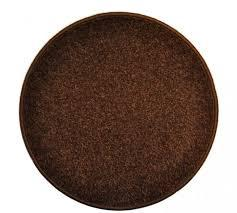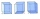# Cylinder - h2

Cylinder volume is 2.6 liters. Base area is 1.3 dm2. Calculate the height of the cylinder.

Correct result:

h =  2 dm

#### Solution:We would be pleased if you find an error in the word problem, spelling mistakes, or inaccuracies and send it to us. Thank you!Tips to related online calculators
Do you know the volume and unit volume, and want to convert volume units?

## Next similar math problems:

• Surface and volumeCalculate the surface and volume of a cylinder whose height is 8 dm and the radius of the base circle is 2 dm
• Tank and waterCylindrical tank were poured with 3.5 liters of water. If tank base diameter is 3 dm, how height is water level in?
• Rotating coneCalculate the volume and the surface area of a rotating cone of base radius r = 2.3 dm and a height h = 46 mm.
• The potThe pot is in 1/3 filled with water. Bottom of the pot has an area of ​​329 cm2. How many centimeters rises water level in the pot after add 1.2 liters of water?
• Tin with oilTin with oil has the shape of a rotating cylinder whose height is equal to the diameter of its base. Canned surface is 1884 cm2. Calculate how many liters of oil is in the tin.
• Volume and surfaceCalculate the volume and surface area of the cylinder when the cylinder height and base diameter is in a ratio of 3:4 and the area of the cylinder jacket is 24 dm2.
• Cylinder - hCylinder volume is 215 cm3. Base radius is 2 cm. Calculate the height of the cylinder.
• PrismThe volume of tetrahedral prism is 2.43 m3. Base of prism is a parallelogram in which a side 2,5dm and height ha = 18cm. Calculate the height of the prism.
• ConeCircular cone with height h = 29 dm and base radius r = 3 dm slice plane parallel to the base. Calculate distance of the cone vertex from this plane, if solids have the same volume.
• Pyramid a+hCalculate the volume and surface area of the pyramid on the edge and height a = 26 cm. h = 3 dm.
• Cylinder - basicsCylinder with base radius r = 45 dm and height h=22 dm. Calculate:
• PrismCalculate the height of the prism having a surface area 448.88 dm² wherein the base is square with a side of 6.2 dm. What will be its volume in hectoliters?
• Square prismCalculate the volume of a square prism of high 2 dm wherein the base is: rectangle with sides 17 cm and 1.3 dm
• CalculateCalculate the surface of a regular quadrilateral prism whose base edge is 2.4dm and the height of the prism is 38cm.
• Cylinder - areaThe diameter of the cylinder is one-third the length of the height of the cylinder. Calculate the surface of cylinder if its volume is 2 m3.
• Cylinder containerThe cylindrical container with a diameter of 1.8 m contains 2,000 liters of water. How high does the water reach?
• Surface areaCalculate the surface area of a four-sides 2-m high prism which base is a rectangle with sides 17 cm and 1.3 dm